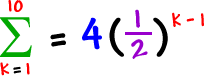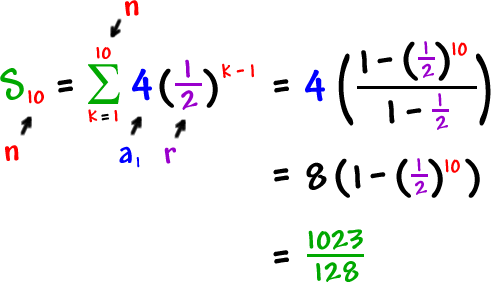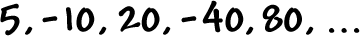So, what if we want to find this sum?Luckily, there's a formula!

 To find the sum of the first n terms of a geometric sequence:Let's use it:Check to make sure the formula works by adding these up:TRY IT:

Find the sum of the first 15 terms of